# 基于无监督学习的自编码器实现

#### 无监督学习介绍及部分函数实现

1. 内容简介

目前许多有监督学习算法，如SVM，DNN或是boosting，决策树等，都在工业界分类或决策任务上取得了不错的成果。但是这些有监督学习需要大量带标签的数据，对数据进行上标签又是一个需耗费人力与时间的任务。有许多数据都是不带标签的，因此我们可利用无监督学习对其进行聚类或特征提取。利用无监督学习得到的特征结果也可应用到带标记数据较少的有监督学习任务中，提高其分类性能。

这门课程，我们将介绍基于无监督学习的一个简单应用——

 自编码器 ，一个可用于降维或者提取特征的神经网络。

2. 实验原理

无监督学习的目的是利用无标记数据推断出数据内部隐藏的结构特征。与有监督学习从有标记的数据中学到一个有正确答案的模型不同，由于无监督学习的数据是未经标记的，因此在学习过程中就没有所谓误差或者指导信号去评估一个可能的解决方案。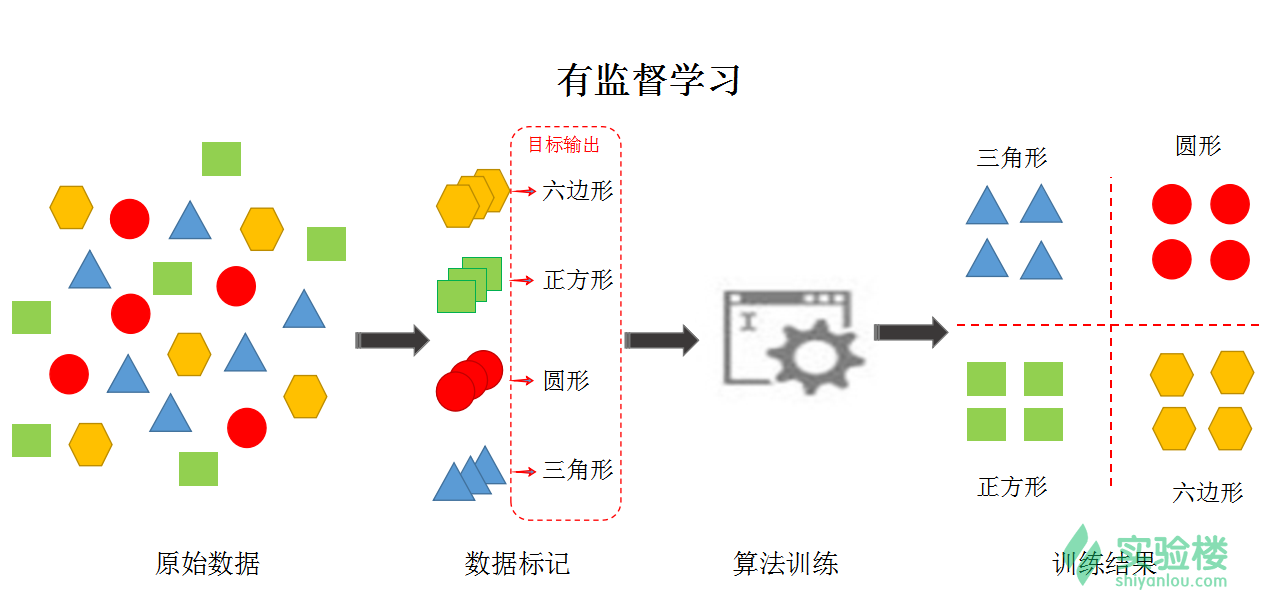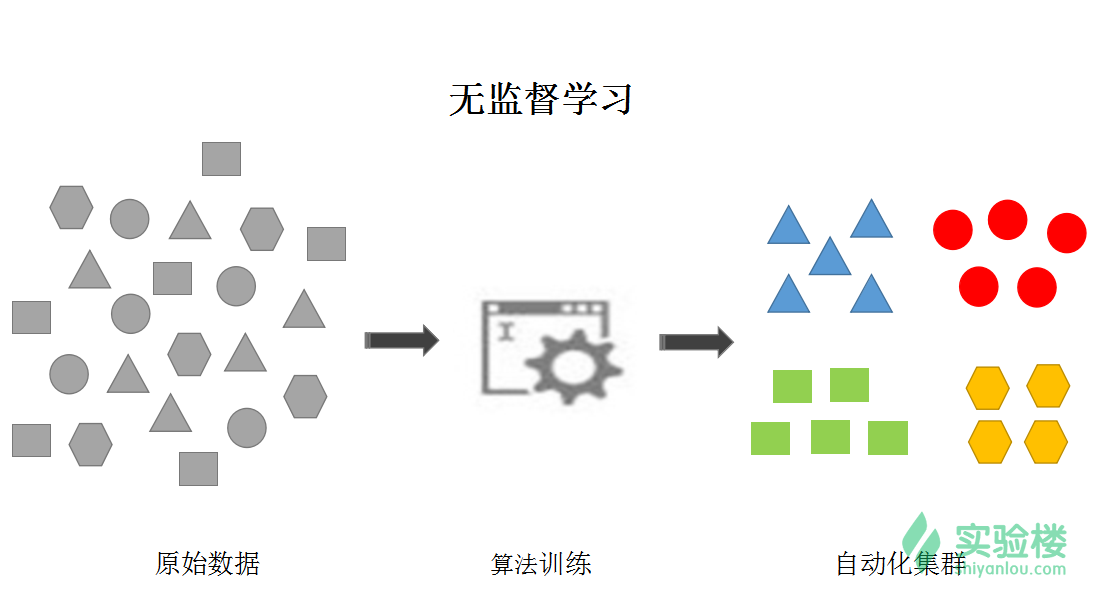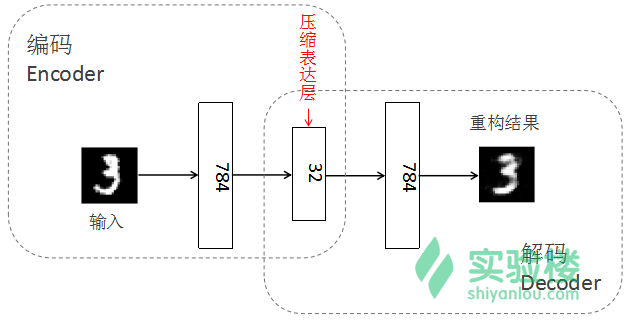hw,b(x)=x
, 即通过自编码器的训练后，
decoder(encoder(x))=x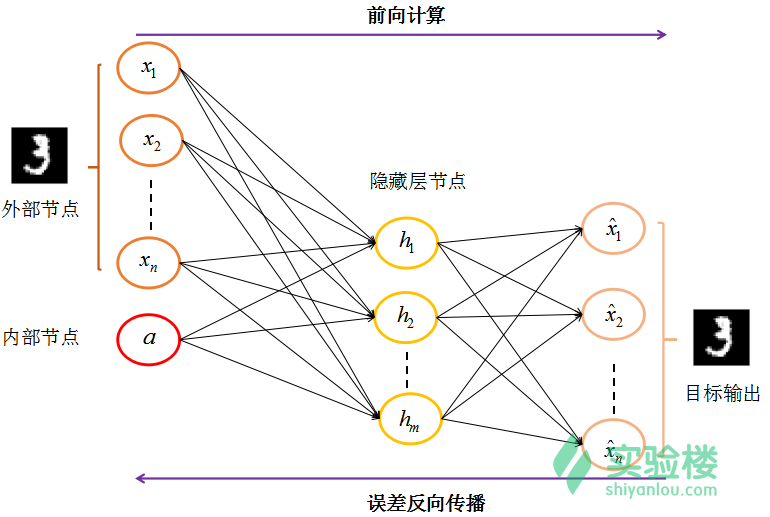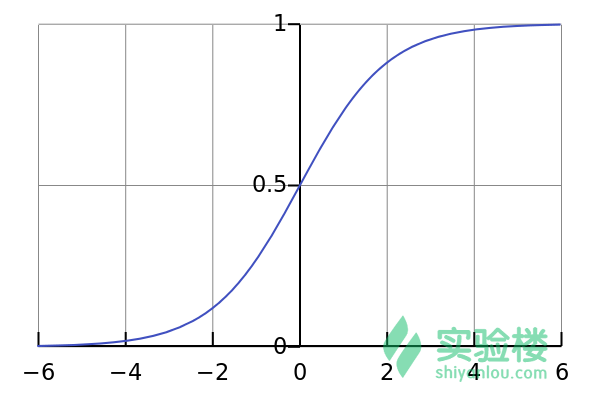hi=f(zhi)

zhi=j=1nxjwj,i+awn+1,j

xi^=f(zi^)

zi^=j=1mwj,ihj

C=1/2i=1n(xi^y)2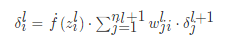#### 自编码器实现

1. 实验过程

数据预处理
在实验过程中，我们用一个列向量表示一个图片样本，如数字图片维度为28*2828∗28时，我们用784*1784∗1的列向量表示其数据，这个过程称为向量化数据。由于输入灰度图片像素值范围在0~255，为加快网络的训练收敛，且原始图像数据由于是0-255的unit数据为便于python里的浮点型运算，我们将图像数据归一化至0-1区间。
这里我们采用的归一化方法为最小-最大标准化（Min-Max Nomalization），转换函数如下：

x=(xmin)/(maxmin)

由于这里图像数据的最大值为255，最小值为0，故在实验过程中，我们归一化时直接将原始数据除以255即可。


1. 监督学习
2. 无监督学习
3. 强化学习

1. 离线学习 / 批梯度下降
2. 在线学习 / 随机梯度下降
3. 最小批梯度下降

​​ ，当根据代价函数计算所得的误差小于某个 任意小的ϵ,ϵ>0ϵ,ϵ>0$\epsilon, \epsilon>0ϵ,ϵ>0$时停止训练。

#-*- coding=utf8 -*-

import scipy.io as scio
import numpy as np
import matplotlib.pyplot as plt
import random

def main():

unlabeled_data = trainData['trainData']
unlabeled_data = unlabeled_data[:,:] / 255.

# define the learning parameters
alpha = 5 # learning rate
max_epoch = 500 # the learning epoch
mini_batch = 100 # used for the batch learning
imgSize = 784 # image size of a digit image in the minist dataset

# the network structure
layer_struc = [[imgSize, 1],
[0, 32],
[0, imgSize]]
layer_num = 3

# initialize weights
w = []
for l in range(layer_num-1):
w.append(np.random.randn(layer_struc[l+1],sum(layer_struc[l])))

dataset_size = 500  # the number of the images in the training data set

# define the internal input of the network
X = []
X.append(np.array(unlabeled_data[:,:]))
X.append(np.zeros((0,dataset_size)))
X.append(np.zeros((0,dataset_size)))

delta = []
for l in range(layer_num):
delta.append([])

# define the display parameters
nRow = max_epoch / 100 + 1
nColumn = 10   # display 10 images in each row
eachDigitNum = 50  # 50 instancese corresponding to each digit in the training set

# display the original digit in the first row

for iImg in range(nColumn):
ax = plt.subplot(nRow, nColumn, iImg+1)
plt.imshow(unlabeled_data[:,eachDigitNum * iImg + 1].reshape((28,28)).T, cmap= plt.cm.gray)

if iImg == 0:
plt.ylabel('Original Images',rotation=90)

ax.get_xaxis().set_visible(False)
ax.get_yaxis().set_visible(False)

# unsupervised training
count = 0 # count the iteration
print('Autoencoder training start..')
for iter in range(max_epoch):

# define the shuffle index
ind = list(range(dataset_size))
random.shuffle(ind)

a = []
z = []
z.append([])
for i in range(int(np.ceil(dataset_size / mini_batch))):
a.append(np.zeros((layer_struc, mini_batch)))
x = []
for l in range(layer_num):
x.append( X[l][:,ind[i*mini_batch : min((i+1)*mini_batch, dataset_size)]])

y = unlabeled_data[:,ind[i*mini_batch:min((i+1)*mini_batch,dataset_size)]]
for l in range(layer_num-1):
a.append([])
z.append([])
a[l+1],z[l+1] = feedforward(w[l],a[l],x[l])

delta[layer_num-1] = np.array(a[layer_num-1] - y) * np.array(a[layer_num-1])
delta[layer_num-1] = delta[layer_num-1] * np.array(1-a[layer_num-1])

for l in range(layer_num-2, 0, -1):
delta[l] = backprop(w[l],z[l],delta[l+1])

for l in range(layer_num-1):
dw = np.dot(delta[l+1], np.concatenate((a[l],x[l]),axis=0).T) / mini_batch
w[l] = w[l] - alpha * dw

count = count + 1

# display reconstruction result
if np.mod(iter+1,100) == 0 :
b = []
b.append(np.zeros((layer_struc,dataset_size)))

for l in range(layer_num-1):
tempA, tempZ = feedforward(w[l], b[l], X[l])
b.append(tempA)

for iImg in range(nColumn):
ax = plt.subplot(nRow,nColumn, iImg + nColumn * (iter+1)/100 + 1)
dis_result = b[layer_num-1][:,eachDigitNum * iImg + 1].reshape(28,28).T
plt.imshow(dis_result,cmap= plt.cm.gray)
ax.get_xaxis().set_visible(False)
ax.get_yaxis().set_visible(False)

print('Learning epoch:', count, '/', max_epoch)

plt.show()

# feedforward computing
def feedforward(w,a,x):
f = lambda s: 1 / (1 + np.exp(-s))

# concatenate the matrix a and x , and multiply with weight matrix
w = np.array(w)
temp = np.array(np.concatenate((a,x),axis=0))
z_next = np.dot(w , temp)

return f(z_next), z_next

# backpropagation
def backprop(w,z,delta_next):

# sigmoid function
f = lambda s: np.array(1 / (1 + np.exp(-s)))

# the Derivative of sigmoid function
df = lambda s: f(s) * (1 - f(s))

delta = df(z) * np.dot(w.T,delta_next)

return delta

if __name__ == '__main__':
main()